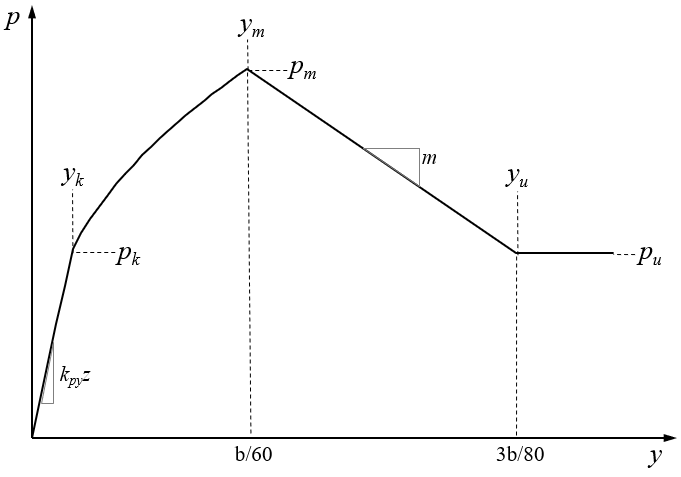# Silt (Cemented C-Phi Soil)

The following parameters are required for calculating the p-y curve for silt:

• Cohesion
• Friction Angle
• Initial Stiffness, kpy

Since the stress-strain behaviour of c-phi soils is closer to that of sand than cohesive soil, the same procedure as used for sand (Reese et al., 1974) is used.p-y curve for Silt